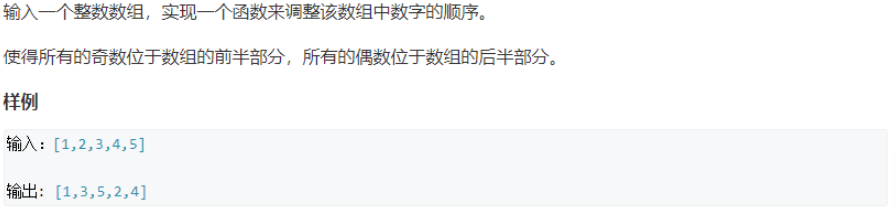# 输入一个整数数组，实现一个函数来调整该数组中数字的顺序，使得所有的奇数位于数组的前半部分，所有的偶数位于位于数组的后半部分，并保证奇数和奇数，偶数和偶数之间的相对位置不变。

+关注继续查看

1. 如果头指针指向的数组位置为奇数，那么就判断尾指针指向的数组位置的奇偶性。如果是奇数，则头指针后移一个位置，如果是偶数，则尾指针前移一个位置。

2. 如果头指针指向的数组位置为偶数，那么就判断尾指针指向的数组位置的奇偶性。如果是奇数，则交换头尾指针指向的数组元素，如果是偶数，则尾指针前移一个位置。

public class Q13_ReOrderArray {

public static void main(String[] args) {
int []array = {1,2,3,4,5,6,7,8,9,10,11,12,13};
reOrderArray(array);
for(int i=0;i<array.length;i++)
{
System.out.print(array[i]+" ");
}
}
public static void reOrderArray(int []array)
{
int i=0;
int j=array.length-1;
while(i<j)
{
if(array[i]%2==1)//奇数
{
if(array[j]%2==1)//奇数
{
i++;
}else//偶数
{
j--;
}
}else//偶数
{
if(array[j]%2==1)//奇数
{
//交换array[i]和array[j]
int temp = array[i];
array[i] = array[j];
array[j] = temp;
}else//偶数
{
j--;
}
}
}
}
}

public class q13_reOrderArray {

public static void main(String[] args) {
int []array = {2,4,6,1,3,5,7};
reOrderArray2(array);
for(int i=0;i<array.length;i++)
{
System.out.print(array[i]+" ");
}
}
public static void reOrderArray2(int []array)
{
int j=0;//记录第一个为奇数的位置
int m=0;//记录排好序的奇数的最后一个位置
for(int i=0;i<array.length;i++)
{
if(array[i]%2==1)//找到第一个奇数
{
int temp = array[i];//记录第一个奇数
int ti=i;
for(;ti>0;ti--)
{
array[ti]=array[ti-1];//将第一个奇数之前的所有元素往后移一个位置
}
array = temp;//将第一个奇数放到array位置
j=i;
break;
}
}
for(++j;j<array.length;j++)//依次寻找剩余的奇数
{
if(array[j]%2==1)
{
int temp = array[j];
int tj = j;
for(;tj>m;tj--)
{
array[tj]=array[tj-1];
}
array[++m]=temp;
}
}

}
}【脚趾 Offer 21 】调整数组顺序使奇数位于偶数前面
【脚趾 Offer 21 】调整数组顺序使奇数位于偶数前面
0 00 00 0AcWing 32. 调整数组顺序使奇数位于偶数前面
AcWing 32. 调整数组顺序使奇数位于偶数前面
0 00 02174 0[剑指offer] 调整数组顺序使奇数位于偶数前面

850 0671 0【37】调整数组顺序使奇数位于偶数前面

1123 0607 0

java程序员